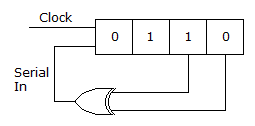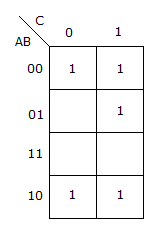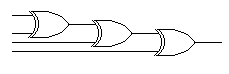# Test: Digital Electronics - 8

## 25 Questions MCQ Test Digital Electronics | Test: Digital Electronics - 8

Description
This mock test of Test: Digital Electronics - 8 for Electrical Engineering (EE) helps you for every Electrical Engineering (EE) entrance exam. This contains 25 Multiple Choice Questions for Electrical Engineering (EE) Test: Digital Electronics - 8 (mcq) to study with solutions a complete question bank. The solved questions answers in this Test: Digital Electronics - 8 quiz give you a good mix of easy questions and tough questions. Electrical Engineering (EE) students definitely take this Test: Digital Electronics - 8 exercise for a better result in the exam. You can find other Test: Digital Electronics - 8 extra questions, long questions & short questions for Electrical Engineering (EE) on EduRev as well by searching above.
QUESTION: 1

Solution:
QUESTION: 2

Solution:
QUESTION: 3

### In standard TTL the totem pole stage refers to

Solution:
QUESTION: 4

The initial contents of the 4 bit serial in parallel out right shift, shift register shown in figure is 0110. After three clock pulses are applied, the contents of the shift register will beSolution:
QUESTION: 5

In 8085 microprocessor the value of the most significant bit of the result following the execution of any arithmetic or Boolean instruction is stored in

Solution:
QUESTION: 6

With 2's complement representation, the range of values that can be represented on the data bus of an 8 bit microprocessor is given by

Solution:
QUESTION: 7

Which of the following correctly declares a pointer to an array of intergers in C?

Solution:
QUESTION: 8

In a modem digital computer, a subtractor in normally not used because

Solution:
QUESTION: 9

In which one of the following types of analog to digital convertors the conversion time is practically independent of the amplitude of the analog signal?

Solution:
QUESTION: 10

In 8085 microprocessor, which of the following is not vectored interrupt?

Solution:
QUESTION: 11

A twisted ring counter consisting of 4 FF will have

Solution:
QUESTION: 12

A clock signal driving a 6-bit ring counter has a frequency of 1 MHz. How long is each timing bit high?

Solution:
QUESTION: 13

A two-input OR gate is designed for positive logic. However, it is operated with negative logic. The resulting logic operation will then be

Solution:
QUESTION: 14

Assertion (A): In a serial in-serial out shift register, access is available only to the left most or right most flip flops

Reason (R): If the output of a shift register is feedback to serial input it can be used as a ring counter.

Solution:
QUESTION: 15

IC counters are

Solution:
QUESTION: 16

2's complement representation of a 16 bit number (one sign bit and 15 magnitude bits) is FFFF. Its magnitude in decimal representation is

Solution:
QUESTION: 17

The method used to transfer data from I/O units to memory by suspending the memory-CPU data transfer for one memory cycle is called

Solution:
QUESTION: 18

Out of SAM and RAM

Solution:
QUESTION: 19

Bipolar IC memories are fabricated using

Solution:
QUESTION: 20

For K map of the given figure the simplified Boolean expression isSolution:
QUESTION: 21

Choose the appropriate turn on and turn off time of a FET

Solution:
QUESTION: 22

The circuit of the given figure isSolution:
QUESTION: 23

Consider the following statements

1. ECL has least propagation delay
2. TTL has largest fan out
3. CMOS has highest noise margin
4. TTL has lowest power dissipation
Which of these are correct?
Solution:
QUESTION: 24

In Schottky TTL, a Schottky diode is used for

Solution:
QUESTION: 25

Which of the following ICs has only one NAND gate?

Solution: